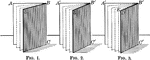### Equal Dihedral Angles

Illustration of 3 equal dihedral angles. "Two dihedral angles have the same ratio as their plane angles."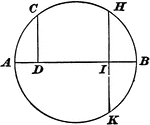### Circle With a Perpendicular Drawn to the Diameter

"If from any point on the circumference of a circle, a perpendicular be let fall upon a given diameter,…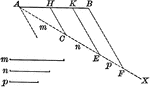### Line Divided Into Parts

Line divided into proportional parts.### Parallel Lines Cut by Transversals

Illustration that shows if two parallel lines are cut by three or more transversals that pass through…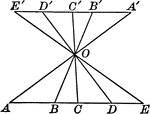### Parallel Lines Cut by Transversals

Illustration that shows if two parallel lines are cut by three or more transversals that pass through…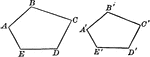### Similar Polygons (Pentagons) That Can Be Used To Show Proportionality

Illustration that shows similar polygons (pentagons) that can be used to show proportionality.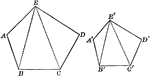### Similar Polygons (Pentagons) That Can Be Used To Show Proportionality

Illustration that shows similar polygons (pentagons) that can be used to show proportionality.### 2 Octagonal Prisms

Illustration of 2 right octagonal prisms with congruent bases, but different heights. The height of…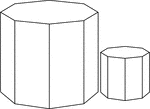### 2 Similar Octagonal Prisms

Illustration of 2 Similar right octagonal prisms. The height and length of the edges of the smaller…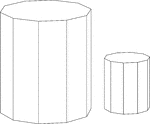### Similar Decagonal Prisms

Illustration of 2 similar right decagonal prisms. Both have regular decagons for bases and rectangular…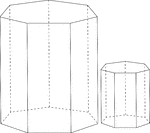### Similar Heptagonal/Septagonal Prisms

Illustration of 2 similar right heptagonal/septagonal prisms. Both have regular heptagons/septagons…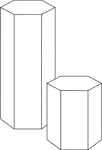### Similar Hexagonal Prisms

Illustration of 2 similar right hexagonal prisms. The height of the prism and length of the side of…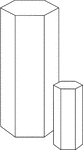### Similar Hexagonal Prisms

Illustration of 2 similar right hexagonal prisms. The height of the prism and length of the side of…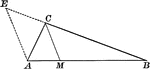### Angle Bisector of Triangle Dividing Opposite Side Into Proportionate Segments

Illustration to show that an angle bisector of a triangle divides the opposite side into segments which…### Exterior Angle Bisector of Triangle Dividing Opposite Side Into Proportionate Segments

Illustration to show that a bisector of a an exterior angle of a triangle divides the opposite side…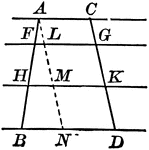### Parallel Lines Cut by Parallels, Corresponding Intercepts Proportional

Illustration to show that if parallel lines are cut by any number of parallels, the corresponding intercepts…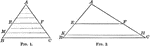### Triangle Divided by Parallel Lines to Form Proportionate Triangles

Illustration to show that if a line is drawn through two sides of a triangle parallel to the third side,…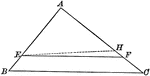### Triangle Divided by Parallel Lines to Form Proportionate Triangles

Illustration to show that if a line divides two sides of a triangle proportionally, it is parallel to…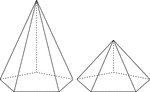### 2 Right Pentagonal Pyramids

Illustration of 2 right pentagonal pyramids with hidden edges shown. The pentagonal bases are congruent,…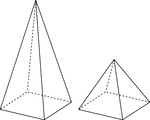### 2 Right Rectangular Pyramids

Illustration of 2 right rectangular pyramids with hidden edges shown. The rectangular bases are congruent,…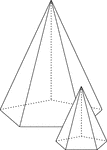### Similar Pentagonal Pyramids

Illustration of 2 similar right pentagonal pyramids with hidden edges shown. The height of the pyramid…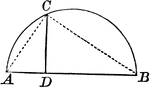### Right Triangle Inscribed in Semicircle Shows Mean Proportional

Right triangle inscribed in semicircle. Illustration shows that the perpendicular from any point in…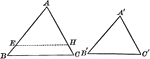### Mutually Equiangular Similar Triangles

Illustration that shows two mutually equiangular triangles are similar.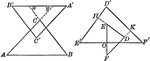### Similar Triangles With Respective Sides Parallel or Perpendicular

Illustration that shows that two triangles which have their sides respectively parallel, or respectively…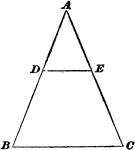### Similar Triangles

Illustration of a triangle divided by a parallel line to form two similar triangles.### Similar Triangles With Altitudes Drawn

Illustration that shows two similar triangles with altitudes drawn.